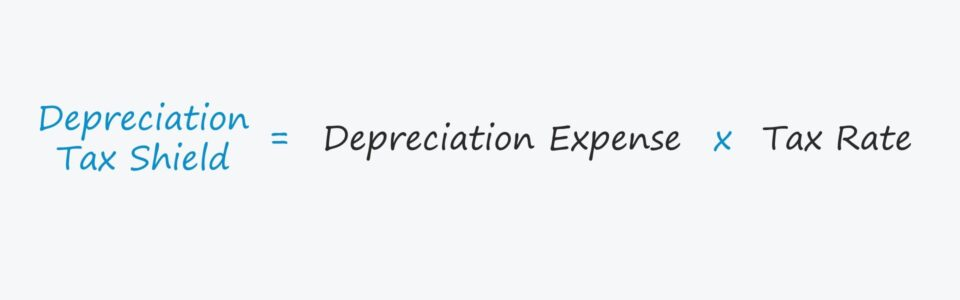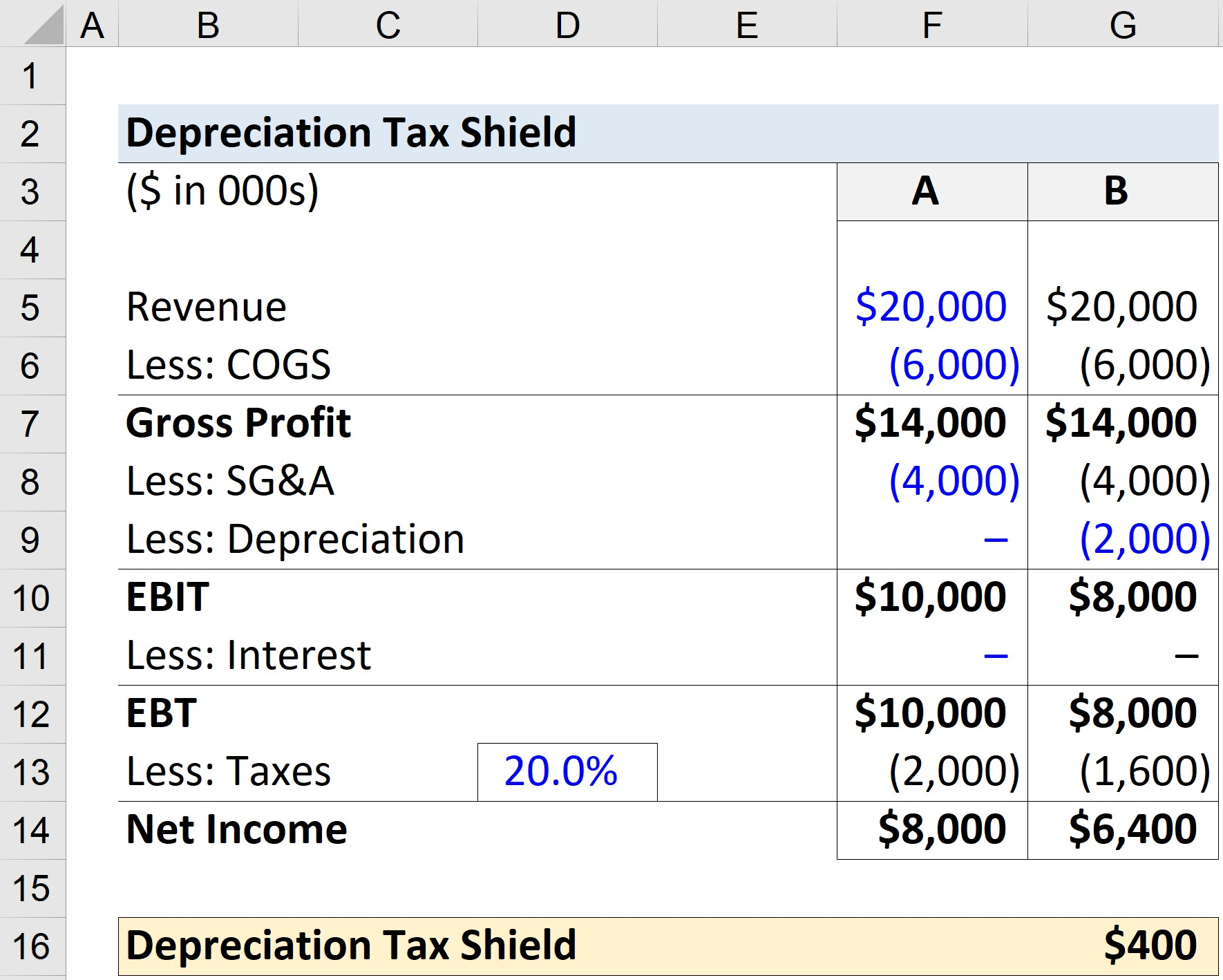Welcome to Wall Street Prep! Use code at checkout for 15% off.# Depreciation Tax Shield

Guide to Understanding the Depreciation Tax Shield## Depreciation Tax Shield: How Depreciation Impacts Taxes

Under U.S. GAAP, depreciation reduces the book value of a company’s property, plant, and equipment (PP&E) over its estimated useful life.

Depreciation expense is an accrual accounting concept meant to “match” the timing of the fixed assets purchases — i.e. capital expenditures — with the cash flows generated from those assets over a period of time.

The real cash outflow stemming from capital expenditures has already occurred, however in U.S. GAAP accounting, the expense is recorded and spread across multiple periods.

The recognition of depreciation causes a reduction to the pre-tax income (or earnings before taxes, “EBT”) for each period, thereby effectively creating a tax benefit.

Those tax savings represent the “depreciation tax shield”, which reduces the tax owed by a company for book purposes.

## How to Calculate Depreciation Tax Shield (Step-by-Step)

In order to calculate the depreciation tax shield, the first step is to find a company’s depreciation expense.

D&A is embedded within a company’s cost of goods sold (COGS) and operating expenses, so the recommended source to find the total value is the cash flow statement (CFS).

Once found, the next step is to copy the D&A value and then search for it in the search box, assuming that the amortization expense was combined with depreciation.

The actual separate depreciation value should be relatively straightforward to find within the SEC filings of the company (or if private, the specific amount might need to be requested from company management if not explicitly provided).

In the final step, the depreciation expense — typically an estimated amount based on historical spending (i.e. a percentage of Capex) and management guidance — is multiplied by the tax rate.

## Depreciation Tax Shield Formula

The formula for calculating the depreciation tax shield is as follows.

Depreciation Tax Shield = Depreciation Expense × Tax Rate %

If feasible, annual depreciation expense can be manually calculated by subtracting the salvage value (i.e. the remaining asset value at the end of its useful life) from the asset’s purchase price, which is subsequently divided by the estimated useful life of the fixed asset.

Because depreciation expense is treated as a non-cash add-back, it is added back to net income on the cash flow statement (CFS).

Therefore, depreciation is perceived as having a positive impact on the free cash flows (FCFs) of a company, which should theoretically increase its valuation.

## Depreciation Tax Shield Calculator

We’ll now move to a modeling exercise, which you can access by filling out the form below.#### Get the Excel Template!Submitting...

## Depreciation Tax Shield Calculation Example

Suppose we are looking at a company under two different scenarios, where the only difference is the depreciation expense.

Under both scenarios — A and B — the financials of the company are as follows:

Income Statement Data:

Therefore, the company’s gross profit equals \$14 million.

• Gross Profit = \$20 million — \$6 million

For Scenario A, the depreciation expense is set to be zero, whereas the annual depreciation is assumed to be \$2 million under Scenario B.

• Scenario A:
• Depreciation = \$0 million
• EBIT = \$14 million – \$4 million = \$10 million
• Scenario B:
• Depreciation = \$2 million
• EBIT = \$14 million – \$4 million – \$2 million = \$8 million

The difference in EBIT amounts to \$2 million, entirely attributable to the depreciation expense.

Since we assume the interest expense to be zero, EBT equals EBIT.

As for the taxes owed, we’ll multiply EBT by our 20% tax rate assumption, and net income is equal to EBT subtracted by the tax.

• Scenario A:
• Taxes = \$10 million * 20% = \$2 million
• Net Income = \$10 million – \$2 million = \$8 million
• Scenario B:
• Taxes = \$8 million * 20% = \$1.6 million
• Net Income = \$8 million – \$1.6 million = \$6.4 million

In Scenario B, the taxes recorded for book purposes is \$400k lower than under Scenario A, reflecting the depreciation tax shield.

• Depreciation Tax Shield = \$2 million – \$1.6 million = \$400kStep-by-Step Online Course

### Everything You Need To Master Financial Modeling

Enroll in The Premium Package: Learn Financial Statement Modeling, DCF, M&A, LBO and Comps. The same training program used at top investment banks.